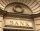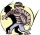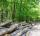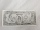# Common ratio

If 200 units of a commodity are consumed in a first year, and if the annual rate of increase in consumption is 5%

(a) what amount is consumed in the 8th year;
(b) in the first 15 years?

a8 =  281.4201
s15 =  4315.7127

### Step-by-step explanation:Did you find an error or inaccuracy? Feel free to write us. Thank you!Tips to related online calculators
Do you want to convert time units like minutes to seconds?

## Related math problems and questions:

• Present valueA bank loans a family $90,000 at 4.5% annual interest rate to purchase a house. The family agrees to pay the loan off by making monthly payments over a 15 year period. How much should the monthly payment be in order to pay off the debt in 15 years? • SavingsThe depositor regularly wants to invest the same amount of money in the financial institution at the beginning of the year and wants to save 10,000 euros at the end of the tenth year. What amount should he deposit if the annual interest rate for the annua • Annual interestA loan of 10 000 euro is to be repaid in annual payments over 10 years. Assuming a fixed 10% annual interest rate compounded annually, calculate: (a) the amount of each annual repayment (b) the total interest paid. • The crimeThe crime rate of a certain city is increasing by exactly 7% each year. If there were 600 crimes in the year 1990 and the crime rate remains constant each year, determine the approximate number of crimes in the year 2025. • Future valueSuppose you invested$1000 per quarter over a 15 years period. If money earns an anual rate of 6.5% compounded quarterly, how much would be available at the end of the time period? How much is the interest earn?
• PopulationWhat is the population of the city with 3% annual growth, if in 10 years the city will have 60,000 residents?
• BankPaul put 10000 in the bank for 6 years. Calculate how much you will have in the bank if he not pick earned interest or change deposit conditions. The annual interest rate is 3.5%, and the tax on interest is 10%.
• Wood in the forestThe amount of wood in the forest was estimated at 6000 m3. How much wood will be in forest after 2 years if the annual growth of wood is 2.5% each year and logging 30 m3 each year?
• The city 2Today lives 298000 citizens in the city. How many citizens can we expect in 8 years if their annual increase is 2.4%?
• Volume of woodEvery year, at the same time, an increase in the volume of wood in the forest is measured. The increase is regularly p% compared to the previous year. If in 10 years the volume of wood has increased by 10%, what is the number p?
• Annual pensionCalculate the amount of money generating an annual pension of EUR 1000, payable at the end of the year and for a period of 10 years, shall be inserted into the bank to account with an annual interest rate of 2%
• Retirement annuityHow much will it cost to purchase a two-level retirement annuity that will pay $2000 at the end of every month for the first 10 years, and$3000 per month for the next 15 years? Assume that the payment represent a rate of return to the person receiving th
• Car valueThe car loses value 15% every year. Determine a time (in years) when its price will halve.
• Saving 9An amount of $2000 is invested at an interest of 5% per month. If$ 200 is added at the beginning of each successive month but no withdrawals. Give an expression for the value accumulated after n months. After how many months will the amount has accumula
• Geometric progressionIn geometric progression, a1 = 7, q = 5. Find the condition for n to sum first n members is sn≤217.
• InflationOnce upon a time, tsar owned a money printer and printed and printed. The result of printing money prices went up,in the first year 3.9 %, in the second 6%, in the third 4.7% and in the fourth 5.5%. Then tsar was failed in election. Calculate the average
• LoanApply for a \$ 59000 loan, the loan repayment period is 8 years, the interest rate 7%. How much should I pay for every month (or every year if paid yearly). Example is for practise geometric progression and/or periodic payment for an annuity.